PHYSICS

Mass

MASS

MA. Fundamental of Mass MB. Kong Frequency and Kong Wavelength MC. Annihilation and Pair Production

MD. Kong Equation

ME. Kong Atom Model MF. Quantum of Atom MG. Perturbation of Photon

MH. Periodic Table

MI. Chemical Reaction MJ. Superconductor MK. Particles and Waves ML. Nuclear Physics

MD. KONG EQUATION

1. Kong Equation 2. Time Dependent Kong Equation 3. Time Independent Kong Equation

4. Discussion on Kong Equation

INTRODUCTION

As described in the chapter “Fundamental of Mass”, currently, the Schrödinger equation is used to define the quantum number for atom. The Kong equation suggests an alternative method in developing the quantum properties and the quantum number of atom complete with proper derivations and solutions.

This chapter only shows the solution of the Kong equation. The application of the Kong equation will be discussed in the chapter “Kong Atom Model” and “Quantum of Atom”. The Kong equation shows more convincing solutions to all the quantum mechanics of atom, and also to the chemical properties and reactions of atoms. An atom model is brief here for pre-understanding.

OBJECTIVES

1)    To derive and solve the Kong equation in developing the quantum properties and the quantum number of the atoms.

2)      To discuss on the solutions of the Kong equation.

3)      To brief on the atom structure.

MD.1.0        KONG EQUATION

The Kong equation plays an important role in solving the quantum mechanics of the atom and all the properties and characteristic of the atom. It is divided into two parts which consists of the time dependent and time independent equation. The Time Dependent Kong Equation gives solutions to the all the quantum properties of atom and the Time Independent Kong Equation gives solutions to the 3 quantum numbers of atom.

The quantum properties of atom will be developed in detail in the chapter “Kong Atom Model”. They are the

1) velocity of electrons,

3) magnetic gauss line of atom,

5) magnetic dipole moment of electron,

4) orbital angular momentum of atom,

6) state energy of atom.

The 3 quantum numbers will be developed in detail in the chapter “Quantum of Atom”. They are the

1) Principle Orbital Number (PON),

2) Sub-Orbital Number (SON),

3) Electron Number (EN).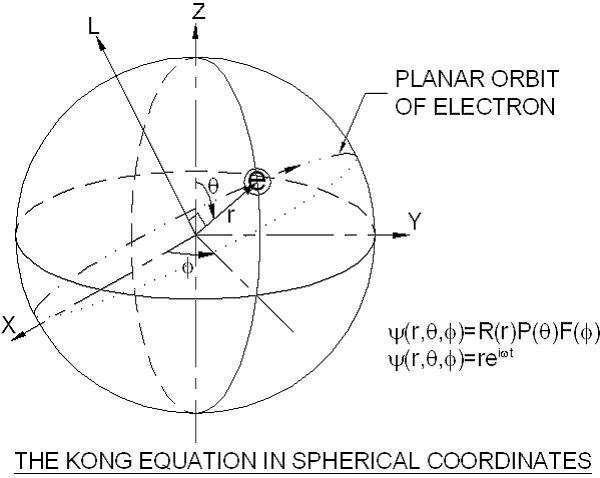Figure MD.1.1

For an electron orbits in an atom in anti-clockwise direction as shown in figure MD.1.1, the function is given as follow,

y = x = r sin f = reif = reiwt                                                             … eq. MD.1.1

For an electron orbits in an atom in clockwise direction, the function is given as follow,… eq. MD.1.2

where   x = distance from origin

Φ = angle from x-axis to the position of electron

ω = angular velocity

t = time

Φ = ωt

The function of the orbiting electron is purely planar so that the electron returns to the same position every cycle. The force acting to the electron is always towards the center of the atom. This planar motion function of electron forms the basic equation for the derivations of the Kong Equation.

MD.2.0        TIME DEPENDENT KONG EQUATION

From eq. MD.1.1, the second order differential time dependent Kong equation is given as follows,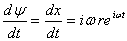… eq. MD.2.1

Rearrange eq. MD.2.1, for an electron circulates on a planar orbit with radius r, the acceleration acts on the electron is given as follow,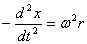… eq. MD.2.2

Eq. MD.2.2 shows the balance of inward and outward forces. The centrifugal acceleration is balanced by the revolution of electron at radius r at angular frequency of w. Eq. MD.2.2 is defined as follows,

Acceleration of Electric force = Acceleration of Magnetic force             … eq. MD.2.3

The forces acting on the electron are the magnetic and electric (M&E) forces. The balance of these two forces causes the electrons orbit in the atom without collapse. Also due to this phenomenon, electrons are able and easy to change position or energy level upon perturbation. The left side of eq. MD.2.2 is the electric force where the force is always pulling the electrons inward to the center of atom. The right side of eq. MD.2.2 is the generated magnetic force caused by the traveling effect when electrons move in the magnetic field at certain velocity. The Hall Effect prevents the electron from being pulled in by electric force. The motion of electron is purely a planar motion and this complies to the Hall Effect, where the magnetic field is perpendicular to the movement of the electrons.

From eq. MD.2.2, we replace the inward centrifugal force with the electric force and the outward force with the magnetic force as follow,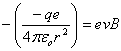… eq. MD.2.4

where   e = electron charge

q = charge of atom

εo = permittivity constant

v = electron velocity =

B = magnetic field

From eq. MD.2.4, the velocity of the electron is,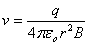… eq. MD.2.5

From eq. MD.2.5, we can conclude that if the orbiting radius, the charge of atom and the magnetic field are constant, the velocity of the electron is constant. The velocity plays important role in developing the energy level of atom. This will be discussed more in the chapter “Kong Atom Model”.

From the above, the Time Dependent Kong Equation shows the force balancing for an orbiting electron in an atom. Therefore, electron does not emit electromagnetic (EM) wave and electron orbits the atom perpetually provided that without external disturbances.

MD.3.0        TIME INDEPENDENT KONG EQUATION

The Time Independent Kong Equation gives the solution of all the quantum numbers of atom. The 3-dimensional Cartesian and Spherical polar coordinates of Time Independent Kong Equation takes the form of,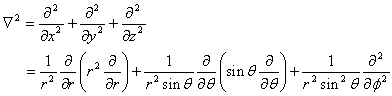… eq. MD.3.1

Substituting the function of orbiting electron (eq. MD.1.1) to the above equation, it forms,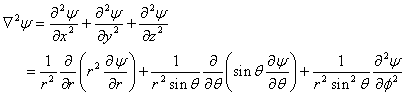… eq. MD.3.2

The Cartesian coordinates produces the following,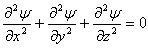… eq. MD.3.3

Substitute eq. MD3.3 into eq. MD.3.2, we obtain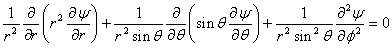… eq. MD.3.4

Rearrange eq. MD.3.4 and multiply by the factor of (r2 sin2q), we obtain the following,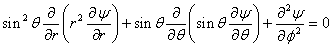… eq. MD.3.5

To solve the above second degree partial differential equation, the function is separated to individual variables, where,

y(r,q,f) = R(r)P(q)F(f)                                                                      … eq. MD.3.6

Substitute eq. MD.3.6 into eq. MD.3.5 and divide by the function, we obtain,… eq. MD.3.7

Eq. MD.3.7 is the Time Independent Kong Equation in spherical polar coordinates.

MD.3.1        AZIMUTHAL EQUATION

Upon separation of the Kong equation, the second order differential azimuthal equation is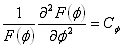… eq. MD.3.8

where Cf = constant

From eq. MD.1.1, the function of F(f) gives the data of,

F(f) = Reif                                                                                          … eq. MD.3.9

Solving eq. MD.3.8 through integration of df twice as follows,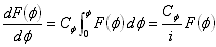Cf = -1                                                                                                 … eq. MD.3.10

For the electron circulates on the same orbit and returns to the same position after each revolution, the azimuthal function is expressed as follow,

F(f) = F(f + 2np) = Rei(f+2np) = Reif + Rei2np                                 … eq. MD.3.11

where   n = integer value for the number of revolutions

The second term on the right side of eq. MD.3.11 can be expanded as follow,

Rei2np = R sin(2np)+ i cos(2np) = Rn sin(2p) = 0                              … eq. MD.3.12

Therefore, the azimuthal equation only produces one solution which is stated in eq. MD.3.10, but not as stated in the Schrödinger equation.

Similarly, substituting the function of eq. MD.1.2 for the clock-wise direction into eq. MD.3.8, where

F(f) = -Reif                                                                                         … eq. MD.3.13

The azimuthal equation for the function of eq. MD.3.13 gives the solution of

Cf = 1                                                                                                  … eq. MD.3.14

Eq. MD.3.10 and eq. MD.3.14 show the solutions of the azimuthal equation and the value of Cf = ±1.

MD.3.2        COLATITUDE EQUATION

From eq. MD.1.1, the function of P(q) gives the no data on the colatitude angle. To proceed with the solution, we first assume that the function of P(q) as follow,

P(q) = Reif = Cq                                                                                … eq. MD.3.15

where Cq = constant

The differentiation of constant gives the value of zero. Therefore, upon separation of the Kong equation, the second order differential colatitude equation is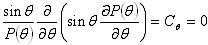… eq. MD.3.16

Upon separation of the Kong equation, the second order differential radial equation is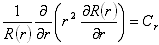… eq. MD.3.17

where Cr = constant

From eq. MD.1.1, the function of R(r) gives the data of,

R(r) = Reif =                                                                                           … eq. MD.3.18

Solving eq. MD.3.17 through integration of dr as follows,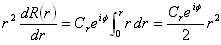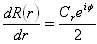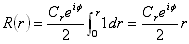… eq. MD.3.19

Eliminate the variable (eif) at both sides, and quantize the radius, eq. MD.3.19 reduces to a function of,

re = n ½rB                                                                                            … eq. MD.3.20

where   Cr = n = principle orbital number = 1, 2, 3, …, ∞

re = Traveling radius of electron

From the hydrogen atom model, at the minimum quantized radius or the first principle orbital number, the radius is given by the Bohr radius, rB. re is the traveling radius of electron. From eq. MD.3.20, one electron travels half of the quantized radius. Therefore one quantized radius can allow for two orbiting electrons. In another expression, one orbit can allow for two electrons.

Multiply number of 2 at both sides of eq. MD.3.20, we obtain,

2re = n rB

ra = n rB                                                                                               … eq. MD.3.21

where   ra = 2re = radius of atom

MD.3.4        Solving Time Independent Kong Equation

From eq. MD.3.7, quoted as follows,… eq. MD.3.7

Substitute the solved constants of each partial differential equation, we obtain

n sin2q + 0 ± 1 = 0                                                                             … eq. MD.3.22

where   Cr = n

The second order differential colatitude equation = 0

Cf = ±1

First, let Cf = 1, we obtain,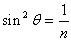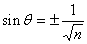… eq. MD.3.23

where   n = principle orbital number = 1, 2, 3, …, ∞

The angle θ shows the allowable tilting angle of the function ψ in an atom at different orbital. Solving the value of θ for different value of n, the results are shown in table MD.3.1 below for the first few principle orbital numbers,

 n sin θ θ 1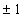2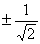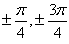3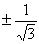4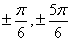5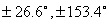6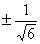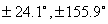7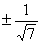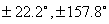8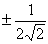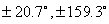Table MD.3.1

From table MD.3.1, it shows that at higher principle orbit number, the allowable tilting angle of orbit is increasing. It creates more space to house the electrons.

Similarly, let Cf = 1, we obtain,… eq. MD.3.24

Eq. MD.3.24 provides imaginary solution which shows that it is impossible. Therefore, the application of eq. MD.1.2 is void. This shows that the electron only travel in anti-clockwise direction, but never in clock-wise direction.

MD.4.0        DISCUSSIONS ON KONG EQUATION

By solving the Time Dependent and Time Independent Kong Equation, it gives many extra information and understanding about the atom structure. These are the pre-understanding of the solution of Kong equation. They shall be read in conjunction with the “Quantum of Atom” and the “Kong Atom Model” for better visualization. Below are the explanations and descriptions base on the solutions of the Kong equation. A brief on the atom structure is presented for pre-study on the descriptions below.

MD.4.1        Electrons Travel In Uni-Direction

From the azimuthal equation, the constant Cf  gives the value of positive and negative unity. However, in solving the Kong equation, it is discovered that the positive unit of Cf  produces imaginary solution. The negative unit of Cf  gives solutions to Kong equation. Here, we can conclude that electrons can only travel in one direction which is the anti-clockwise direction.

MD.4.2        Number of Electrons per Orbit

From the "Pair Production", it is derived that electron possesses the spin angular momentum which is half of the angular momentum of atom. The angular momentum of atom will be derived in the next chapter "Kong Atom Model". From eq. MD.3.20 and eq. MD.3.21, two electrons circulate on an orbit make a single full rotation. Therefore, only two electrons are allowed in each orbit.

MD.4.3        Hall Effect

The solution of Time Dependent Kong Equation tells that electrons are force balance between the electric and magnetic forces. Therefore, electrons do not emit electromagnetic wave. The uni-directional movement of electrons confirmed that the electrons never collapse into the atom due to the Hall Effect. Otherwise, electrons will collapse if circulate in the clockwise direction.

MD.4.4        Non-Clashing of Electrons

Electrons have the same quantized charge. Electrons which travel on the same orbit and circulate at same velocity have the same energy level. Each orbit can house for two electrons that travel at the same velocity. Therefore, electrons do not clash with each other.

MD.4.5        Balance of Angular Momentum

In the atom structure, two electrons orbit with the angle of p apart each other. The center of rotation is the center of atom. The uni-directional circulation of the two electrons confirms the system (atom) is momentously balance.

MD.4.6        Tilting Effect

For the principle orbit number higher than 1 and above, more than one sub-orbitals are allowed. The tilting of sub-orbital is due to the repulsive force of electrons. When more than three electrons are allowed at the same principle orbital number, two electrons fill within the same sub-orbit; the third electron fills another sub-orbital. The repulsive force of electrons at different sub-orbital tends to push away from each other and causes the electron to tilt from the horizontal axis. Due to this tilting effect of electrons, molecules produce all kinds of structure, such as molecule H2O possesses 'V' shape.

MD.4.7        Atom Structure

Figure MD.4.1 suggests the front view of a helium atom with two orbiting electrons. It shows the magnetic gauss line acts as the orbit to house the electrons. The magnetic gauss line penetrates the center of the electrons and the magnetic dipole moment is parallel to the direction of the magnetic gauss line. Electron has up spin and the intrinsic spin magnetic dipole moment is downward, obeying the direction of the magnetic gauss line of the atom.

The atom is a charged particle. The atom originally possesses its own magnetic moment of ma. When electrons circulate in anti-clockwise direction, the moving charge particles of electrons produces magnetic moment me, which directionally opposite to ma and 'neutralize' ma in order to stabilize the atom. If the electrons circulate in planar perpendicular to the direction of ma, two circulating electrons produce equal magnetic moment, me that balances off the atom magnetic moment, ma. Therefore, the atom is said magnetically neutral.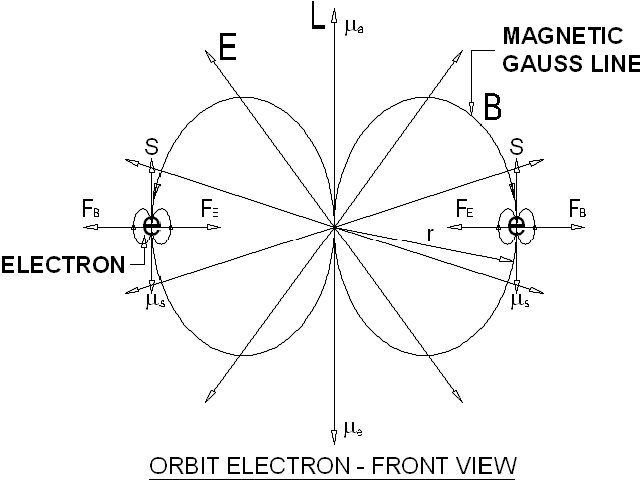Figure MD.4.1Figure MD.4.2

Figure MD.4.2 shows the top view of the same atom. The electrons circulate on the magnetic gauss line in anti-clockwise direction when viewing on top. The circulation of electrons at certain velocity produces magnetic field. The resultant magnetic field creates a magnetic force outward, balance off the inward electric force. The Hall Effect prevents the electrons from collapsing.

DISCUSSIONS AND CONCLUSIONS

Electron circulates in planar shape where it returns to the original position after each revolution. This is the basis for the development of Kong Equation. The Time Dependent Kong Equation provides the solutions to all the quantum properties of atom. The Time Independent Kong Equation provides the solutions to the quantum numbers of atom.

Electrons travel in uni-direction in atom. The circulation of electrons without collapsing into atom is due to the force balance between the two magnetic and electric forces. Two half spin electrons circulate on the same orbit at same direction in achieving a full spin of atom. One orbit can only house for maximum two electrons.

Two electrons are separated apart with the angle of p. The atom is momentously balance where the center of rotation of the two electrons is the center of atom. The separated electrons travel at the same velocity on the same orbit prevent clashes with each other. At higher principle orbit number, electron orbits are tilted at certain angle due to the repulsive force of the electrons.

This website is originated on 15-Mar-2007,

updated on 4-Jan-2009.